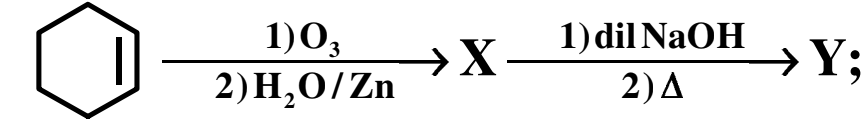Y is

#Y is

1. A
2. B
3. C
4. D

Fill Out the Form for Expert Academic Guidance!l

+91

Live ClassesBooksTest SeriesSelf Learning

Verify OTP Code (required)

### Solution:

The 1st step of the reaction is ozonolysis of cyclohexene. so, X will be adipaldehyde. The next step of the reaction is aldol condensation. adipaldehyde reacts with dil. $\mathrm{NaOH}$ and form aldol product cyclopent-1-ene-1-carbaldehyde.

Hence, the correct option (B) cyclopent-1-ene-1-carbaldehyde is correct option.

## Related content

 Differences & Comparisons Articles in Chemistry Magnesium oxide Formula Barium chloride formula Benzoic Acid formula Butane Formula Magnesium Chloride Formula Magnesium hydroxide Formula Nitrite Formula Phosphoric Acid Formula Salicylic Acid Formula+91

Live ClassesBooksTest SeriesSelf Learning

Verify OTP Code (required)